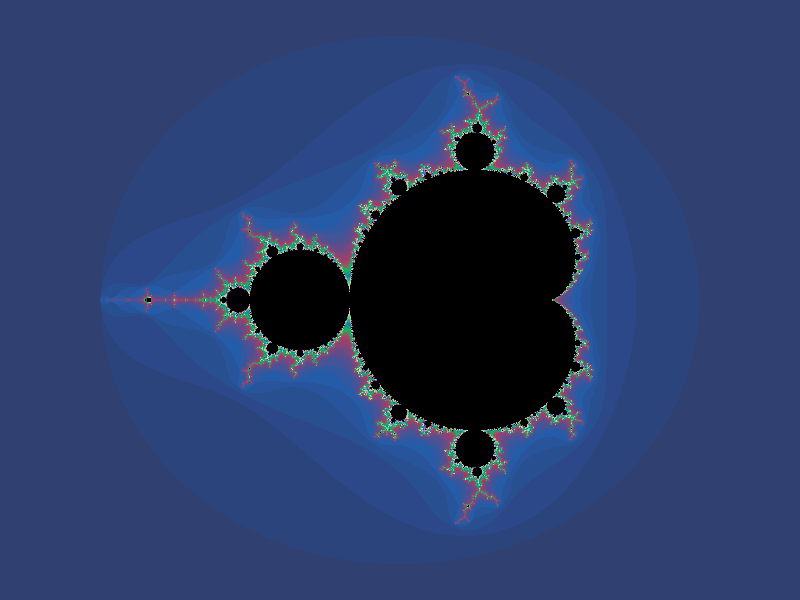#Fractals

Math Lair Home > Topics > Fractals

While fractals are complex shapes, some perceive them as being too complicated to understand, but that's not really the case.Fractals are all about repetition. Some start with a basic shape such as a line or a triangle, changed to make a few new (smaller) copies, and repeated to any level of detail.

One of the earliest fractals, although it wasn't known as such at the time, was defined by the German mathematician Georg Cantor and is known as the Cantor Set. To create this, start with a straight line segment and remove the middle third of the line, leaving you with two smaller line segments. Repeat the process with each of the two smaller line segments, and so on. Another early fractal is the Koch curve, which appeared in a paper by Helge von Koch in 1904. To create it, start with a straight line, replace the middle third of each line with a triangle, and repeat the process indefinitely. Here are the results of the first few iterations:The Sierpinski Gasket results from starting with a square and removing the central 1/3 × 1/3 square.

The name "fractal" comes from the fact that they have fractional dimension. This means that magnifying a fractal shows more perimeter or area than would be expected from a "normal" shape. There are several ways of determining a fractal's dimension. The simplest (although one that doesn't work with every fractal) is the similarity dimension. Take a look at the Koch curve above. If you were to magnify it by a factor of 3, you would get 4 exact copies of it. So, its fractal dimension would be (log 4)/(log 3), which equals approximately 1.26.

No matter how much you magnify a fractal, there is always more detail that can never be completely resolved. They have visual irregularities that, when you magnify them, reveal complexity as great as what was apparent at the beginning.

The picture at the top of the page is a picture of the Mandelbrot set, one of the most famous fractals. This fractal is named after Benoit Mandelbrot, who produced images of this set in the 1970s. The actual set consists of the points plotted in black. The colour values represent how quickly a point goes to infinity when iterated; black points never reach infinity. If you were to look at this set under greater and greater magnification, you would see more detail that can't be seen in this picture.

One of the uses of fractals is that they can serve as tools for analyzing a wide range of natural phenomena, such as the shapes of mountain ranges (an early application of this can be found in the movie Star Trek II: The Wrath of Khan), coastlines, rivers, trees, and clouds. Mandelbrot discovered that these phenomena are statistically self-similar; while they are not exactly self-similar like many fractals are, as you zoom in on these phenomena, a similar pattern is produced. For example, the pattern of branching found in a small branch of a tree is similar to the pattern of branching in the larger branches.

In the natural world, there can be a great difference in the measurement of an object depending on the size of the yardstick. This is because, as you zoom, more detail is revealed, like a fractal. For example, if you were to look at the coastline of a lake from the space shuttle and measure it, you might find the length of the coastline to be, say, 50 kilometres. If you were to fly over it in an airplane, you would notice tiny bays and coves and indentations and the like that weren't visible from outer space, and you might measure the coastline to be, say, 200 kilometres. If you were to walk along the shoreline, walking around every indentation, you would find the length of the coastline to be even longer. Zooming in even further, if an ant were to walk along the coastline, walking around every grain of sand that forms the border between the shore and the water, the ant would have to walk thousands and thousands of kilometres.